Mathematical and Physical Journal
for High Schools
Issued by the MATFUND Foundation
 Already signed up? New to KöMaL?

# KöMaL Problems in Physics, September 2012

Show/hide problems of signs:## Problems with sign 'M'

Deadline expired on October 10, 2012.

M. 325. Charge a capacitor of known capacitance (e.g. 2200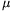F) to a given voltage (e.g. 9 V), and then discharge it through a resistor of known resistance (e.g. 47 k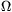). Measure and plot the graph of the voltage across the condenser as a function of the elapsed time. How long does it take for the voltage to decrease to

a) one half;

b) one fourth of its initial value?

(6 pont)

statistics## Problems with sign 'P'

Deadline expired on October 10, 2012.

P. 4455. A body rolling along a horizontal surface reaches a slope. (The slope joins the horizontal smoothly.) In which case will the body get higher, if there is friction or if there is no friction? Reason why.

(3 pont)

solution (in Hungarian), statistics

P. 4456. A liquid in glass thermometer, which is at room temperature initially, is put to warm water, and it is observed that the level of the liquid in the thermometer initially decreases one or two degrees, before it begins to increase.

What can the reason of this behaviour be?

(3 pont)

solution (in Hungarian), statistics

P. 4457. A one end of a thread of lengthis tied to a small iron ball, whilst the other end is fixed at a height ofabove a tabletop. The ball is displaced such that the thread is horizontal and is kept at this position by an electromagnet. At a height ofabove the tabletop another small iron ball, similar to the one at the end of the thread, is also kept by an electromagnet. The two electromagnets release the balls at the same moment. The two balls begin to move at the same instant.

Which one reaches the tabletop first?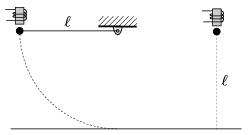(4 pont)

solution (in Hungarian), statistics

P. 4458. Three young men would like to go to the disco club, which is at a distance of 8.8 km. The speed of the bicycle with one person is 20 km/h, with two people it is 16 km/h. Three people cannot go together on one bicycle, and their speed is 4 km/h on foot. How can they all get to the disco club in one hour?

(4 pont)

solution (in Hungarian), statistics

P. 4459. A certain amount of ideal gas contains Helium atoms and Oxygen molecules. The mass of the mixture is 20 gram, its volume is 18.63 litres at a temperature of 27 oC and at a pressure of 200 kPa.

How many moles of Helium and how many moles of Oxygen are there in the gas?

(4 pont)

solution (in Hungarian), statistics

P. 4460. 31.4 J of heat is given to a sample of ideal gas. Because of this the gas expands from a volume of 0.3 litres to 0.8 litres, at a constant pressure of 1.57.104 Pa.

a) Determine the change in the internal energy.

b) What is the degree of freedom of the gas?

(4 pont)

solution (in Hungarian), statistics

P. 4461. What is the relationship between the values of the capacitance of the capacitors of C1, C2,C3, C4, if the equivalent capacitances between the points A and B are the same in both the cases of a) and b)?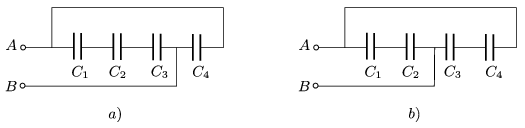(4 pont)

solution (in Hungarian), statistics

P. 4462. A resistor of resistance R=8is operated with the aid of the variable resistor of resistance R0=30in the circuit shown in the figure. The e.m.f. of the voltage supply is U0=24 V, and its internal resistance is negligible.

a) Where is the pointer of the variable resistor when the voltage across the resistor is 12 V?

b) In this case, what percentage of the total dissipated power is the power dissipated in the resistor?

c) Between what values can the dissipated power in the resistor be varied? Between what values can the power given off by the voltage supply vary?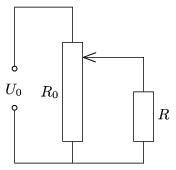(4 pont)

solution (in Hungarian), statistics

P. 4463. Is it true that the energy of any photon of the visible light emitted by an incandescent lamp is greater than the average kinetic energy of an atom of the noble gas which fills the bulb?

Maybe the answer depends on what type of gas is in the bulb?

(4 pont)

solution (in Hungarian), statistics

P. 4464. A certain type of alpha-radiation can penetrate to a depth of 4 cm to a sample of air at a pressure of 105 Pa. To what depth would it penetrate into a sample of less dense air at a pressure of 103 Pa? (The-particle -- being a heavy'' particle -- hardly changes its direction while it interacts with the atoms, so its path is approximately a straight line.)

(5 pont)

solution (in Hungarian), statistics